## A charge of 4.5 × 10-5 C is placed in an electric field with a strength of 2.0 × 104 . If the charge is 0.030 m from the source of the elect

Question

A charge of 4.5 × 10-5 C is placed in an electric field with a strength of 2.0 × 104 . If the charge is 0.030 m from the source of the electric field, what is the electric potential energy of the charge?

in progress 0
7 months 2021-08-08T14:09:58+00:00 1 Answers 8 views 0

## Answers ( )

0.027 J

Explanation:

The formula of the potential energy in electrostatic (U) is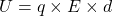The values given in the question are

Charge : q =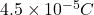Electric Field strength : E =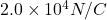Distance : d  is 0.030 m

Insert in the formula , will give us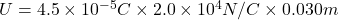Further solving it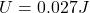Which is the required answer.

Thanks